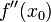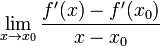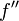# Difference between revisions of "Second derivative"

Jump to: navigation, search

## Definition at a point

### Definition in terms of first derivative

The second derivative of a function$f$ at a point$x_0$, denoted$\! f''(x_0)$, is defined as the derivative at the point$x_0$ of the function defined as the derivative$f'$

Note that the first differentiation operation must be performed, not just at the point, but at all points near it, so that we have a function for the first derivative around the point, which we can then differentiate to calculate the second derivative at the point. It is not good enough to calculate the first derivative only at the particular point (i.e., to calculate only$f'(x_0)$) and then proceed to differentiate that; we need the value of the first derivative at nearby points too.

### Definition as a limit expression

The second derivative of a function$f$ at a point$x_0$, denoted$\! f''(x_0)$, is defined as follows:$\! \lim_{x \to x_0} \frac{f'(x) - f'(x_0)}{x - x_0}$

More explicitly, this can be written as:$\! \lim_{x \to x_0} \frac{1}{x - x_0}\left[\lim_{x_1 \to x} \frac{f(x_1) - f(x)}{x_1 - x} - \lim_{x_2 \to x_0} \frac{f(x_2) - f(x_0)}{x_2 - x_0}\right]$

## Definition as a function

The second derivative of a function at a point is defined as the derivative of the derivative of the function. For a function$f$, the second derivative$f''$ is defined as:$\! f'' := (f')'$